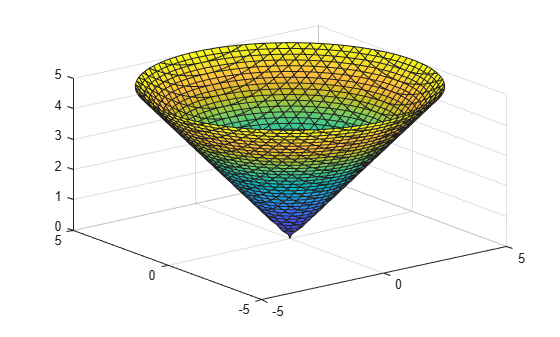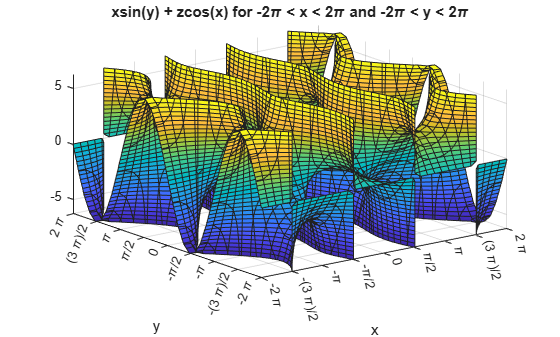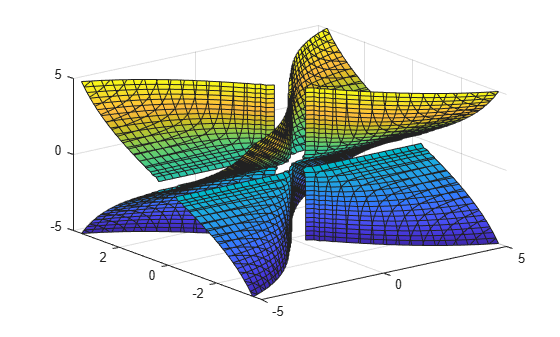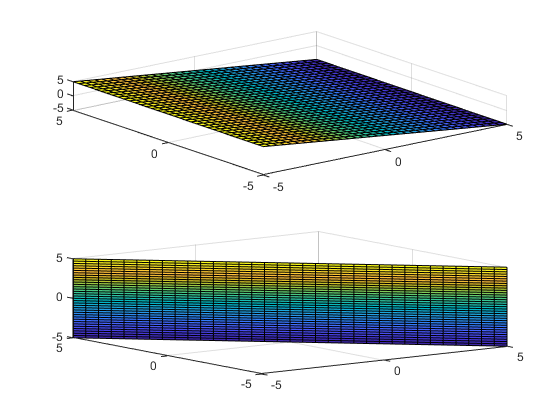Main Content

# fimplicit3

Plot 3-D implicit equation or function

## Syntax

fimplicit3(f)
fimplicit3(f,[min max])
fimplicit3(f,[xmin xmax ymin ymax zmin zmax])
fimplicit3(___,LineSpec)
fimplicit3(___,Name,Value)
fimplicit3(ax,___)
fi = fimplicit3(___)

## Description

example

fimplicit3(f) plots the 3-D implicit equation or function f(x,y,z) over the default interval [-5 5] for x, y, and z.

example

fimplicit3(f,[min max]) plots f(x,y,z) over the interval [min max] for x, y, and z.

example

fimplicit3(f,[xmin xmax ymin ymax zmin zmax]) plots f(x,y,z) over the interval [xmin xmax] for x, [ymin ymax] for y, and [zmin zmax] for z. The fimplicit3 function uses symvar to order the variables and assign intervals.

example

fimplicit3(___,LineSpec) uses LineSpec to set the line style, marker symbol, and face color.

example

fimplicit3(___,Name,Value) specifies line properties using one or more Name,Value pair arguments. Use this option with any of the input argument combinations in the previous syntaxes.
fimplicit3(ax,___) plots into the axes with the object ax instead of the current axes object gca.

example

fi = fimplicit3(___) returns an implicit function surface object. Use the object to query and modify properties of a specific surface. For details, see ImplicitFunctionSurface Properties.

## Examples

collapse all

Plot the hyperboloid ${x}^{2}+{y}^{2}-{z}^{2}=0$ by using fimplicit3. The fimplicit3 function plots over the default interval of $\left[-5,5\right]$ for $x$, $y$, and $z$.

syms x y z fimplicit3(x^2 + y^2 - z^2)Plot the hyperboloid specified by the function $f\left(x,y,z\right)={x}^{2}+{y}^{2}-{z}^{2}$. The fimplicit3 function plots over the default interval of $\left[-5,5\right]$ for $x$, $y$, and $z$.

syms f(x,y,z) f(x,y,z) = x^2 + y^2 - z^2; fimplicit3(f)Specify the plotting interval by specifying the second argument to fimplicit3. Plot the upper half of the hyperboloid ${x}^{2}+{y}^{2}-{z}^{2}=0$ by specifying the interval $0. For $x$ and $y$, use the default interval $\left[-5,5\right]$.

syms x y z f = x^2 + y^2 - z^2; interval = [-5 5 -5 5 0 5]; fimplicit3(f, interval)Plot the implicit equation $x\mathrm{sin}\left(y\right)+z\mathrm{cos}\left(x\right)=0$ over the interval $\left(-2\pi ,2\pi \right)$ for all axes.

Create the x-axis ticks by spanning the x-axis limits at intervals of pi/2. Convert the axis limits to precise multiples of pi/2 by using round and get the symbolic tick values in S. Display these ticks by using the XTick property. Create x-axis labels by using arrayfun to apply texlabel to S. Display these labels by using the XTickLabel property. Repeat these steps for the y-axis.

To use LaTeX in plots, see latex.

syms x y z eqn = x*sin(y) + z*cos(x); fimplicit3(eqn,[-2*pi 2*pi]) title('xsin(y) + zcos(x) for -2\pi < x < 2\pi and -2\pi < y < 2\pi') xlabel('x') ylabel('y') ax = gca; S = sym(ax.XLim(1):pi/2:ax.XLim(2)); S = sym(round(vpa(S/pi*2))*pi/2); ax.XTick = double(S); ax.XTickLabel = arrayfun(@texlabel,S,'UniformOutput',false); S = sym(ax.YLim(1):pi/2:ax.YLim(2)); S = sym(round(vpa(S/pi*2))*pi/2); ax.YTick = double(S); ax.YTickLabel = arrayfun(@texlabel, S, 'UniformOutput', false);Plot the implicit surface ${x}^{2}+{y}^{2}-{z}^{2}=0$ with different line styles for different values of $z$. For $-5, use a dashed line with green dot markers. For $-2, use a LineWidth of 1 and a green face color. For $2, turn off the lines by setting EdgeColor to none.

syms x y z f = x^2 + y^2 - z^2; fimplicit3(f,[-5 5 -5 5 -5 -2],'--.','MarkerEdgeColor','g') hold on fimplicit3(f,[-5 5 -5 5 -2 2],'LineWidth',1,'FaceColor','g') fimplicit3(f,[-5 5 -5 5 2 5],'EdgeColor','none')Plot the implicit surface $1/{x}^{2}-1/{y}^{2}+1/{z}^{2}=0$. Specify an output to make fimplicit3 return the plot object.

syms x y z f = 1/x^2 - 1/y^2 + 1/z^2; fi = fimplicit3(f)fi = ImplicitFunctionSurface with properties: Function: [1x1 sym] EdgeColor: [0 0 0] LineStyle: '-' FaceColor: 'interp' Show all properties 

Show only the positive x-axis by setting the XRange property of fi to [0 5]. Remove the lines by setting the EdgeColor property to 'none'. Visualize the hidden surfaces by making the plot transparent by setting the FaceAlpha property to 0.8.

fi.XRange = [0 5]; fi.EdgeColor = 'none'; fi.FaceAlpha = 0.8;Control the resolution of an implicit surface plot by using the 'MeshDensity' option. Increasing 'MeshDensity' can make smoother, more accurate plots while decreasing 'MeshDensity' can increase plotting speed.

Divide a figure into two by using subplot. In the first subplot, plot the implicit surface $\mathrm{sin}\left(1/\left(xyz\right)\right)$. The surface has large gaps. Fix this issue by increasing the 'MeshDensity' to 40 in the second subplot. fimplicit3 fills the gaps showing that by increasing 'MeshDensity' you increased the resolution of the plot.

syms x y z f = sin(1/(x*y*z)); subplot(2,1,1) fimplicit3(f) title('Default MeshDensity = 35') subplot(2,1,2) fimplicit3(f,'MeshDensity',40) title('Increased MeshDensity = 40')Apply rotation and translation to the implicit surface plot of a torus.

A torus can be defined by an implicit equation in Cartesian coordinates as

$\mathit{f}\left(\mathit{x},\mathit{y},\mathit{z}\right)={\left({\mathit{x}}^{2}+{\mathit{y}}^{2}+{\mathit{z}}^{2}+{\mathit{R}}^{2}-{\mathit{a}}^{2}\right)}^{2}-4{\mathit{R}}^{2}\left({\mathit{x}}^{2}+{\mathit{y}}^{2}\right)$

where

• $a$ is the radius of the tube

• $R$ is the distance from the center of the tube to the center of the torus

Define the values for $a$ and $R$ as 1 and 5, respectively. Plot the torus using fimplicit3.

syms x y z a = 1; R = 4; f(x,y,z) = (x^2+y^2+z^2+R^2-a^2)^2 - 4*R^2*(x^2+y^2); fimplicit3(f) hold on

Apply rotation to the torus around the $x$-axis. Define the rotation matrix. Rotate the torus by 90 degrees or $\pi /2$ radians. Shift the center of the torus by 5 along the $x$-axis.

alpha = pi/2; Rx = [1 0 0; 0 cos(alpha) sin(alpha); 0 -sin(alpha) cos(alpha)]; r = [x; y; z]; r_90 = Rx*r; g = subs(f,[x,y,z],[r_90(1)-5,r_90(2),r_90(3)]);

Add a second plot of the rotated and translated torus to the existing graph.

fimplicit3(g) axis([-5 10 -5 10 -5 5]) hold off## Input Arguments

collapse all

3-D implicit equation or function to plot, specified as a symbolic equation, expression, or function. If an expression or function is specified, then fimplicit3 assumes the right-hand size to be 0.

Plotting interval for x-, y- and z- axes, specified as a vector of two numbers. The default is [-5 5].

Plotting interval for x-, y- and z- axes, specified as a vector of six numbers. The default is [-5 5 -5 5 -5 5].

Axes object. If you do not specify an axes object, then fimplicit3 uses the current axes.

Line style, marker, and color, specified as a character vector or string containing symbols. The symbols can appear in any order. You do not need to specify all three characteristics (line style, marker, and color). For example, if you omit the line style and specify the marker, then the plot shows only the marker and no line.

Example: '--or' is a red dashed line with circle markers

Line StyleDescription
-Solid line
--Dashed line
:Dotted line
-.Dash-dot line
MarkerDescription
'o'Circle
'+'Plus sign
'*'Asterisk
'.'Point
'x'Cross
'_'Horizontal line
'|'Vertical line
's'Square
'd'Diamond
'^'Upward-pointing triangle
'v'Downward-pointing triangle
'>'Right-pointing triangle
'<'Left-pointing triangle
'p'Pentagram
'h'Hexagram
ColorDescription

y

yellow

m

magenta

c

cyan

r

red

g

green

b

blue

w

white

k

black

### Name-Value Pair Arguments

Specify optional comma-separated pairs of Name,Value arguments. Name is the argument name and Value is the corresponding value. Name must appear inside quotes. You can specify several name and value pair arguments in any order as Name1,Value1,...,NameN,ValueN.

Example: 'Marker','o','MarkerFaceColor','red'

The properties listed here are only a subset. For a complete list, see ImplicitFunctionSurface Properties.

Number of evaluation points per direction, specified as a number. The default is 35.

Example: 100

Line color, specified as 'interp', an RGB triplet, a hexadecimal color code, a color name, or a short name. The default RGB triplet value of [0 0 0] corresponds to black. The 'interp' value colors the edges based on the ZData values.

For a custom color, specify an RGB triplet or a hexadecimal color code.

• An RGB triplet is a three-element row vector whose elements specify the intensities of the red, green, and blue components of the color. The intensities must be in the range [0,1]; for example, [0.4 0.6 0.7].

• A hexadecimal color code is a character vector or a string scalar that starts with a hash symbol (#) followed by three or six hexadecimal digits, which can range from 0 to F. The values are not case sensitive. Thus, the color codes '#FF8800', '#ff8800', '#F80', and '#f80' are equivalent.

Alternatively, you can specify some common colors by name. This table lists the named color options, the equivalent RGB triplets, and hexadecimal color codes.

Color NameShort NameRGB TripletHexadecimal Color CodeAppearance
'red''r'[1 0 0]'#FF0000''green''g'[0 1 0]'#00FF00''blue''b'[0 0 1]'#0000FF''cyan' 'c'[0 1 1]'#00FFFF''magenta''m'[1 0 1]'#FF00FF''yellow''y'[1 1 0]'#FFFF00''black''k'[0 0 0]'#000000''white''w'[1 1 1]'#FFFFFF''none'Not applicableNot applicableNot applicableNo color

Here are the RGB triplets and hexadecimal color codes for the default colors MATLAB® uses in many types of plots.

RGB TripletHexadecimal Color CodeAppearance
[0 0.4470 0.7410]'#0072BD'[0.8500 0.3250 0.0980]'#D95319'[0.9290 0.6940 0.1250]'#EDB120'[0.4940 0.1840 0.5560]'#7E2F8E'[0.4660 0.6740 0.1880]'#77AC30'[0.3010 0.7450 0.9330]'#4DBEEE'[0.6350 0.0780 0.1840]'#A2142F'Line style, specified as one of the options listed in this table.

Line StyleDescriptionResulting Line
'-'Solid line'--'Dashed line':'Dotted line'-.'Dash-dotted line'none'No lineNo line

Line width, specified as a positive value in points, where 1 point = 1/72 of an inch. If the line has markers, then the line width also affects the marker edges.

The line width cannot be thinner than the width of a pixel. If you set the line width to a value that is less than the width of a pixel on your system, the line displays as one pixel wide.

Marker symbol, specified as one of the values listed in this table. By default, the object does not display markers. Specifying a marker symbol adds markers at each data point or vertex.

ValueDescription
'o'Circle
'+'Plus sign
'*'Asterisk
'.'Point
'x'Cross
'_'Horizontal line
'|'Vertical line
'square' or 's'Square
'diamond' or 'd'Diamond
'^'Upward-pointing triangle
'v'Downward-pointing triangle
'>'Right-pointing triangle
'<'Left-pointing triangle
'pentagram' or 'p'Five-pointed star (pentagram)
'hexagram' or 'h'Six-pointed star (hexagram)
'none'No markers

Marker outline color, specified as 'auto', an RGB triplet, a hexadecimal color code, a color name, or a short name. The default value of 'auto' uses the same color as the EdgeColor property.

For a custom color, specify an RGB triplet or a hexadecimal color code.

• An RGB triplet is a three-element row vector whose elements specify the intensities of the red, green, and blue components of the color. The intensities must be in the range [0,1]; for example, [0.4 0.6 0.7].

• A hexadecimal color code is a character vector or a string scalar that starts with a hash symbol (#) followed by three or six hexadecimal digits, which can range from 0 to F. The values are not case sensitive. Thus, the color codes '#FF8800', '#ff8800', '#F80', and '#f80' are equivalent.

Alternatively, you can specify some common colors by name. This table lists the named color options, the equivalent RGB triplets, and hexadecimal color codes.

Color NameShort NameRGB TripletHexadecimal Color CodeAppearance
'red''r'[1 0 0]'#FF0000''green''g'[0 1 0]'#00FF00''blue''b'[0 0 1]'#0000FF''cyan' 'c'[0 1 1]'#00FFFF''magenta''m'[1 0 1]'#FF00FF''yellow''y'[1 1 0]'#FFFF00''black''k'[0 0 0]'#000000''white''w'[1 1 1]'#FFFFFF''none'Not applicableNot applicableNot applicableNo color

Here are the RGB triplets and hexadecimal color codes for the default colors MATLAB uses in many types of plots.

RGB TripletHexadecimal Color CodeAppearance
[0 0.4470 0.7410]'#0072BD'[0.8500 0.3250 0.0980]'#D95319'[0.9290 0.6940 0.1250]'#EDB120'[0.4940 0.1840 0.5560]'#7E2F8E'[0.4660 0.6740 0.1880]'#77AC30'[0.3010 0.7450 0.9330]'#4DBEEE'[0.6350 0.0780 0.1840]'#A2142F'Example: [0.5 0.5 0.5]

Example: 'blue'

Example: '#D2F9A7'

Marker fill color, specified as 'auto', an RGB triplet, a hexadecimal color code, a color name, or a short name. The 'auto' value uses the same color as the MarkerEdgeColor property.

For a custom color, specify an RGB triplet or a hexadecimal color code.

• An RGB triplet is a three-element row vector whose elements specify the intensities of the red, green, and blue components of the color. The intensities must be in the range [0,1]; for example, [0.4 0.6 0.7].

• A hexadecimal color code is a character vector or a string scalar that starts with a hash symbol (#) followed by three or six hexadecimal digits, which can range from 0 to F. The values are not case sensitive. Thus, the color codes '#FF8800', '#ff8800', '#F80', and '#f80' are equivalent.

Alternatively, you can specify some common colors by name. This table lists the named color options, the equivalent RGB triplets, and hexadecimal color codes.

Color NameShort NameRGB TripletHexadecimal Color CodeAppearance
'red''r'[1 0 0]'#FF0000''green''g'[0 1 0]'#00FF00''blue''b'[0 0 1]'#0000FF''cyan' 'c'[0 1 1]'#00FFFF''magenta''m'[1 0 1]'#FF00FF''yellow''y'[1 1 0]'#FFFF00''black''k'[0 0 0]'#000000''white''w'[1 1 1]'#FFFFFF''none'Not applicableNot applicableNot applicableNo color

Here are the RGB triplets and hexadecimal color codes for the default colors MATLAB uses in many types of plots.

RGB TripletHexadecimal Color CodeAppearance
[0 0.4470 0.7410]'#0072BD'[0.8500 0.3250 0.0980]'#D95319'[0.9290 0.6940 0.1250]'#EDB120'[0.4940 0.1840 0.5560]'#7E2F8E'[0.4660 0.6740 0.1880]'#77AC30'[0.3010 0.7450 0.9330]'#4DBEEE'[0.6350 0.0780 0.1840]'#A2142F'Example: [0.3 0.2 0.1]

Example: 'green'

Example: '#D2F9A7'

Marker size, specified as a positive value in points, where 1 point = 1/72 of an inch.

## Output Arguments

collapse all

One or more objects, returned as a scalar or a vector. The object is an implicit function surface object. You can use these objects to query and modify properties of a specific line. For details, see ImplicitFunctionSurface Properties.

## Algorithms

fimplicit3 assigns the symbolic variables in f to the x axis, the y axis, then the z axis, and symvar determines the order of the variables to be assigned. Therefore, variable and axis names might not correspond. To force fimplicit3 to assign x, y, or z to its corresponding axis, create the symbolic function to plot, then pass the symbolic function to fimplicit3.

For example, the following code plots the roots of the implicit function f(x,y,z) = x + z in two ways. The first way forces fimplicit3 to assign x and z to their corresponding axes. In the second way, fimplicit3 defers to symvar to determine variable order and axis assignment: fimplicit3 assigns x and z to the x and y axes, respectively.

syms x y z; f(x,y,z) = x + z; figure; subplot(2,1,1) fimplicit3(f); view(-38,71); subplot(2,1,2) fimplicit3(f(x,y,z)); % Or fimplicit3(x + z);## See Also

### Topics

Introduced in R2016b

## Support

#### Mathematical Modeling with Symbolic Math Toolbox

Get examples and videos